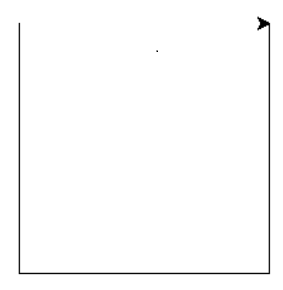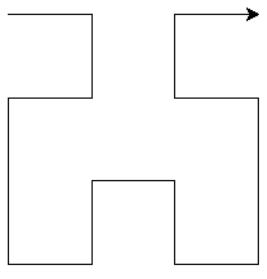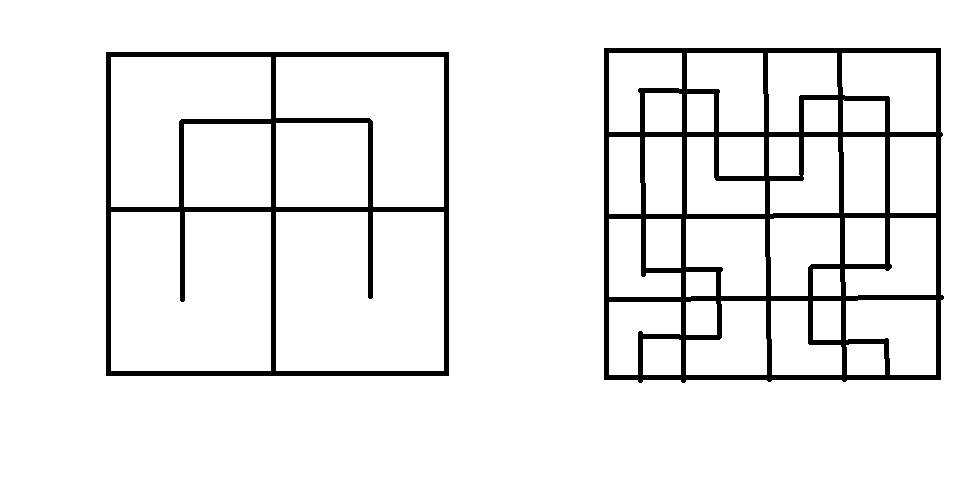Open In App

# Python – Hilbert Curve using turtle

Fractal is a curve or a figure which repeats itself. It comprises a recursive pattern that repeats itself up to a desired level of nesting. Turtle graphics are provided in the turtle module which is used for drawing various shapes and patterns in Python.

A Hilbert curve is a curve that is formed by connecting a sequence of U-shaped curves arranged and oriented in different directions. These U-shaped curves are placed at a certain step size distance apart.

Let us examine a Level-1 Hilbert Curve. The following steps will draw a simple U curve. Let y = 90 degree

1. Rotate y degree towards the right
2. Move step size
3. Rotate y degree towards the left
4. Move step size
5. Rotate y degree towards the left
6. Move step size
7. Rotate y degree towards the rightLet us examine and try to understand the level-2 Hilbert Curve. Again, we assume that the turtle pointer points towards right initially. The following steps may be used to draw the curve:

1. Rotate 90 degrees towards the right
2. Create a hilbert curve at level 1 rotated by -y degrees (ie, y degrees in anticlockwise direction)
3. Move step size
4. Rotate y degrees towards the right
5. Create a level 1 hilbert curve rotated by y degrees (ie, y degrees in clockwise direction)
6. Rotate y degrees towards the left.
7. Move step size
8. Create a level 1 hilbert curve rotated by -y degrees
9. Rotate y degrees towards the rightTurtle methods used in this section are as follows:

• forward(): Used for moving the turtle forward by a given distance in the direction of the turtle.
• backward(): Used for moving the turtle backward by a given distance in the direction of the turtle.
• left(): Used for rotating the turtle in the left direction by a specified angle.
• right(): Used for rotating the turtle in the right direction by a specified angle.
• goto(): Used for moving the turtle to the location specified ((x, y) coordinates).
• penup(): Used for specifying that no drawing will be made while moving.
• pendown(): Used for specifying that drawing will be made while moving.
• done(): Used to specify that the turtle work is completed.

Hibert Curve:

It is also called a peano or space-filling curve. It requires successive approximation. In the first approximation the square is divided into 4 quadrants and draw the curve that connects the center points of each. In the second approximation further, every quadrant is divided which cannot be the center of each.Hibert Curve

• There is no limit to the subdivision. Ideally length of the curve is infinite. With every subdivision the length Increase by 4
• The curve is equivalent to line. Its topological dimension is 1.
• Length of the curve changes by 4.
```N = sDf
4 = 2Df
Df = 2   DT = 1    Df = 2
Df > DT                                    ```

So if a line but folded such that it looks like a 2D object.

• Point sets, curves, and surfaces which has fractal dimension greater than the topological dimension are referred to as fractals.
• The curve generation starts with a square. In a first approximation, the square is divided into 4 quadrants and the curve joins the center of all quadrants by a straight line.
• .In the quadrants it is subdivided into 2*2 grids, ending in 16 squares. Curve visits the center of each small square in each quadrant before moving to the next quadrant
• In 16 squares it is subdivided into 2 * 2 grids, ending in 64 squares. Curve visits the center of each small square before moving to the higher-level quadrant.
• On applying this process continuously,
• The curve never crosses itself
• Curve gets closer to a square containing it
• With each subdivision, the length of the curve increases four times.
• There is no limit on depth and hence there is no limit on curve length.

Applications of Hibert Curve:

• It is used for Modelling natural structures like Geographic terrain, mountain, plant structure, clouds, vegetables etc.
• Space research.
• Study of convergence of iterative processes.
• Engineering and architecture.
• Medical science.
• Chemical processes.
• Medical diagnostic images.
• Fluid mechanics.
• Image compression and different Telecommunication purposes.

Code:

## Python3

 `# Code for Hilbert Curve` `from` `turtle ``import` `*`  `def` `hilbert(level, angle, step):` `    ``# Input Parameters are numeric``    ``# Return Value: None``    ``if` `level ``=``=` `0``:``        ``return` `    ``right(angle)``    ``hilbert(level``-``1``, ``-``angle, step)` `    ``forward(step)``    ``left(angle)``    ``hilbert(level``-``1``, angle, step)` `    ``forward(step)``    ``hilbert(level``-``1``, angle, step)` `    ``left(angle)``    ``forward(step)``    ``hilbert(level``-``1``, ``-``angle, step)``    ``right(angle)`  `def` `main():``    ``level ``=` `int``(``input``())``    ``size ``=` `200``    ``penup()``    ``goto(``-``size ``/` `2.0``, size ``/` `2.0``)``    ``pendown()` `    ``# For positioning turtle``    ``hilbert(level, ``90``, size``/``(``2``*``*``level``-``1``))``    ``done()`  `if` `__name__ ``=``=` `'__main__'``:``    ``main()`